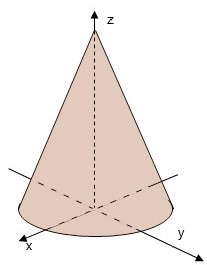# The surface of an island is defined by the function over the region on which the function is...

## Question:

The surface of an island is defined by the function over the region on which the function is nonnegative.

Find the volume of the island.

{eq}z= 25 - \sqrt{x^2 + y^2} {/eq}## Triple Integrals

The volume under a surface {eq}\displaystyle z=f(x,y) {/eq} and above a plane region

{eq}\displaystyle \mathcal{D}=\{(x,y)|\, a\leq x\leq b, u(x)\leq y\leq v(x)\} {/eq} is given as {eq}\displaystyle \iint_{\mathcal{D}}f(x,y)dA {/eq} and evaluated iteratively as

{eq}\displaystyle \int_a^b\int_{u(x)}^{v(x)}f(x,y)\, dydx. {/eq}

If the region to integrate is bounded by a conic, then it is convenient to use polar coordinates

{eq}\displaystyle x=r\cos \theta, y=r\sin \theta, \\ \displaystyle \mathcal{D}=\{(r,\theta)|\, \theta_1\leq \theta\leq \theta_2, u(\theta)\leq r\leq v(\theta)\} {/eq} and the double integral becomes

{eq}\displaystyle \int_{\theta_1}^{\theta_2}\int_{u(\theta)}^{v(\theta)} r\ f(r,\theta) \ dr d \theta. {/eq}

## Answer and Explanation:

The volume of island is the volume under the surface {eq}\displaystyle z=25-\sqrt{x^2+y^2}, {/eq} and above the xy-plane, where the projection of the surface onto the xy- plane is

the disk centered at the origin and radius 25.

Therefore, the volume is written as

{eq}\displaystyle \iint_{\mathcal{D}}\left(25-\sqrt{x^2+y^2}\right) dA {/eq}

The integral in polar coordinate needs the region in polar coordinates {eq}\displaystyle 0\leq \theta\leq 2\pi, 0 \leq r\leq 25, {/eq}

and the integrand function in polar coordinates, {eq}\displaystyle z=25-r. {/eq}

So, the volume is given by

{eq}\displaystyle \begin{align*} \iint_{\mathcal{D}}\left(25-\sqrt{x^2+y^2}\right) dA&= \int_0^{2\pi}\int_{0}^{25} r\ \left(25-r\right)dr d\theta\\ &= \int_0^{2\pi} \ \left(\frac{25}{2}r^2-\frac{1}{3}r^3\right)\bigg\vert_{0}^{25} d\theta\\ &= \int_0^{2\pi} \ \left(\frac{25^3}{2}-\frac{25^3}{3}\right) d\theta\\ &= \frac{25^3}{6}\int_0^{2\pi} d\theta\\ &= \frac{25^3}{6}\cdot 2\pi=\boxed{\frac{25^3\ \pi}{3}}. \end{align*} {/eq}

#### Learn more about this topic:Double Integrals & Evaluation by Iterated Integrals

from GRE Math: Study Guide & Test Prep

Chapter 15 / Lesson 4
401